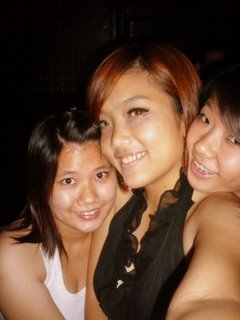## 困

（不好意思拉~本人还在这话题兜圈圈）

+_+_+_+_+_+_+_+_+_+_+_+_+_+_+_+_+_+_+_+_+_+_+_+_+_+_+_+_+_+_+_+_+_+_+_+_+_+_+_+_+_+_+_+_+